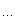# 罗朝辉（飘飘白云）

C++博客 :: 首页 :: 新随笔 :: 联系 :: 聚合:: 管理 ::85 随笔 :: 0 文章 :: 169 评论 :: 0 Trackbacks

CC 许可，转载请注明出处
block 是 Apple 在 GCC 4.2 中扩充的新语法特性，其目的是支持多核并行编程。我们可以将 dispatch_queue 与 block 结合起来使用，方便进行多线程编程。

1，实验工程准备

int main (int argc, const char * argv[])
{
NSAutoreleasePool
* pool = [[NSAutoreleasePool alloc] init];

// insert code hereNSLog(@"Hello, World!");

[pool drain];

return 0;
}

2，如何编写 block

void (^aBlock)(void= ^(void){ NSLog(@"Hello, World!"); };
aBlock();

void (^aBlock)(void= 0;
aBlock
= ^(void) {
NSLog(
@"Hello, World!");
};

aBlock();

void (^blocks)(void= {

^(void){ NSLog(@" >> This is block 1!"); },

^(void){ NSLog(@" >> This is block 2!"); }
};

blocks[
0]();
blocks[
1]();

block 是分配在 stack 上的，这意味着我们必须小心里处理 block 的生命周期。

dispatch_block_t block;

if (x) {
block
= ^{ printf("true\n"); };
}
else {
block
= ^{ printf("false\n"); };
}
block();

if (x) {

struct Block __tmp_1 =// setup details
block = &__tmp_1;
}
else {

struct Block __tmp_2 =// setup details
block = &__tmp_2;
}

3，如何在 block 中修改外部变量

NSAutoreleasePool * pool = [[NSAutoreleasePool alloc] init];

__block
int blockLocal  = 100;

static int staticLocal  = 100;

void (^aBlock)(void= ^(void){
NSLog(
@" >> Sum: %d\n", global + staticLocal);

global
++;
blockLocal
++;
staticLocal
++;
};

aBlock();

NSLog(
@"After modified, global: %d, block local: %d, static local: %d\n", global, blockLocal, staticLocal);

[pool drain];

NSAutoreleasePool * pool = [[NSAutoreleasePool alloc] init];

// 1
void (^aBlock)(int= 0;

static void (^ const staticBlock)(int= ^(int i) {

if (i > 0) {
NSLog(
@" >> static %d", i);
staticBlock(i
- 1);
}
};

aBlock
= staticBlock;
aBlock(
5);

// 2
__block void (^blockBlock)(int);
blockBlock
= ^(int i) {

if (i > 0) {
NSLog(
@" >> block %d", i);
blockBlock(i
- 1);
}
};

blockBlock(
5);

[pool drain];

4，上面我们介绍了 block 及其基本用法，但还没有涉及并行编程。 block 与 Dispatch Queue 分发队列结合起来使用，是 iOS 中并行编程的利器。请看代码：
NSAutoreleasePool * pool = [[NSAutoreleasePool alloc] init];

initData();

// create dispatch queue

//
dispatch_queue_t queue = dispatch_queue_create("StudyBlocks", NULL);

dispatch_async(queue,
^(void) {

int sum = 0;

for(int i = 0; i < Length; i++)
sum
+= data[i];

NSLog(
@" >> Sum: %d", sum);

flag
= YES;
});

// wait util work is done.

//
while (!flag);
dispatch_release(queue);

[pool drain];

dispatch_queue_t 的定义如下：
typedef void (^dispatch_block_t)( void);

dispatch_queue_create 的定义如下：
dispatch_queue_t dispatch_queue_create(const char *label, dispatch_queue_attr_t attr);

dispatch_queue_t dispatch_get_global_queue(long priority, unsigned long flags);

dispatch_async 函数的定义如下：
void dispatch_async(dispatch_queue_t queue, dispatch_block_t block);

5，dispatch_queue 的运作机制及线程间同步

NSAutoreleasePool * pool = [[NSAutoreleasePool alloc] init];

initData();

// Create a semaphore with 0 resource

//
__block dispatch_semaphore_t sem = dispatch_semaphore_create(0);

// create dispatch semaphore

//
dispatch_queue_t queue = dispatch_queue_create("StudyBlocks", NULL);

dispatch_async(queue,
^(void) {

int sum = 0;

for(int i = 0; i < Length; i++)
sum
+= data[i];

NSLog(
@" >> Sum: %d", sum);

// signal the semaphore: add 1 resource

//
dispatch_semaphore_signal(sem);
});

// wait for the semaphore: wait until resource is ready.

//
dispatch_semaphore_wait(sem, DISPATCH_TIME_FOREVER);

dispatch_release(sem);
dispatch_release(queue);

[pool drain];

6 示例：

NSAutoreleasePool * pool = [[NSAutoreleasePool alloc] init];

initData();

__block
int sum = 0;

// Create a semaphore with 0 resource

//
__block dispatch_semaphore_t sem = dispatch_semaphore_create(0);
= dispatch_semaphore_create(0);

// create dispatch semaphore

//
dispatch_queue_t queue = dispatch_queue_create("StudyBlocks", NULL);

= ^(void) {

int s = 0;

for (int i = 0; i < Length; i++)
s
+= data[i];
sum
= s;

NSLog(
@" >> after add: %d", sum);

};

= ^(void) {

int s = sum;

for (int i = 0; i < Length; i++)
s
-= data[i];
sum
= s;

NSLog(
@" >> after subtract: %d", sum);
dispatch_semaphore_signal(sem);
};

// wait for the semaphore: wait until resource is ready.

//
dispatch_semaphore_wait(sem, DISPATCH_TIME_FOREVER);

dispatch_release(sem);
dispatch_release(queue);

[pool drain];

7，使用 dispatch_apply 进行并发迭代：

NSAutoreleasePool * pool = [[NSAutoreleasePool alloc] init];

initData();

dispatch_queue_t queue
= dispatch_get_global_queue(DISPATCH_QUEUE_PRIORITY_DEFAULT, 0);

__block
int sum = 0;
__block
int *pArray = data;

// iterations

//
dispatch_apply(Length, queue, ^(size_t i) {
sum
+= pArray[i];
});

NSLog(
@" >> sum: %d", sum);

dispatch_release(queue);

[pool drain];

dispatch_apply 的定义如下：
dispatch_apply(size_t iterations, dispatch_queue_t queue, void (^block)(size_t));

8, dispatch group

NSAutoreleasePool * pool = [[NSAutoreleasePool alloc] init];

initData();

__block
int sum = 0;

// Create a semaphore with 0 resource

//

// create dispatch semaphore

//
dispatch_queue_t queue = dispatch_queue_create("StudyBlocks", NULL);
dispatch_group_t group
= dispatch_group_create();

= ^(void) {

int s = 0;

for (int i = 0; i < Length; i++)
s
+= data[i];
sum
= s;

NSLog(
@" >> after add: %d", sum);

};

= ^(void) {

int s = sum;

for (int i = 0; i < Length; i++)
s
-= data[i];
sum
= s;

NSLog(
@" >> after subtract: %d", sum);
};

// Fork

// Join
dispatch_group_wait(group, DISPATCH_TIME_FOREVER);

dispatch_release(queue);
dispatch_release(group);

[pool drain];

Concurrency Programming Guide：
posted on 2011-08-26 13:23 罗朝辉 阅读(12266) 评论(5)  编辑 收藏 引用 所属分类: 移动开发Cocoa 开发### 评论

# re: 深入浅出 iOS多线程编程之 block 与 dispatch quene 2011-09-02 01:44 zenny_chen

# re: [Cocoa]深入浅出Cocoa多线程编程之 block 与 dispatch quene 2011-10-31 17:53 hsiun

# re: [Cocoa]深入浅出Cocoa多线程编程之 block 与 dispatch quene 2012-05-19 11:46 tony508
dispatch_queue_create本来不就是串行的，为什么还要加semaphore  回复  更多评论

# re: [Cocoa]深入浅出Cocoa多线程编程之 block 与 dispatch quene 2012-05-23 07:42 罗朝辉
@tony508

# re: [Cocoa]深入浅出Cocoa多线程编程之 block 与 dispatch quene 2012-10-23 22:44 Neu_Tobe